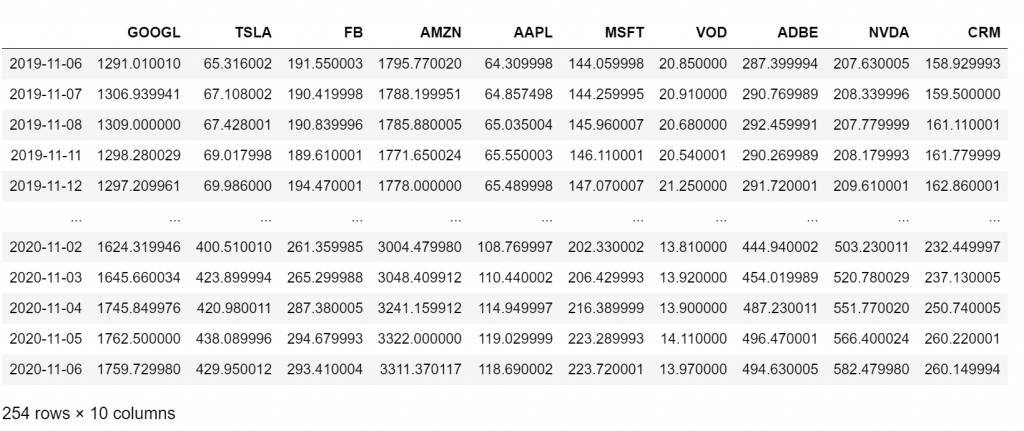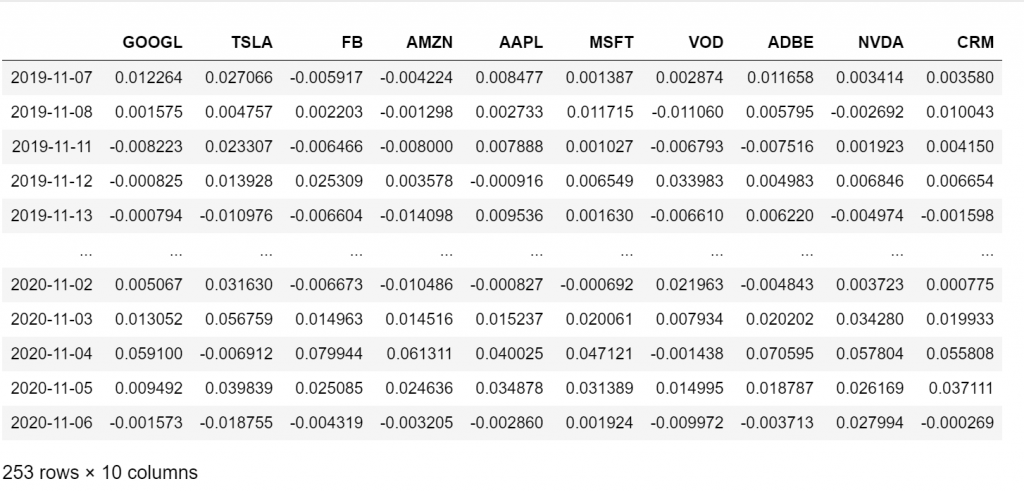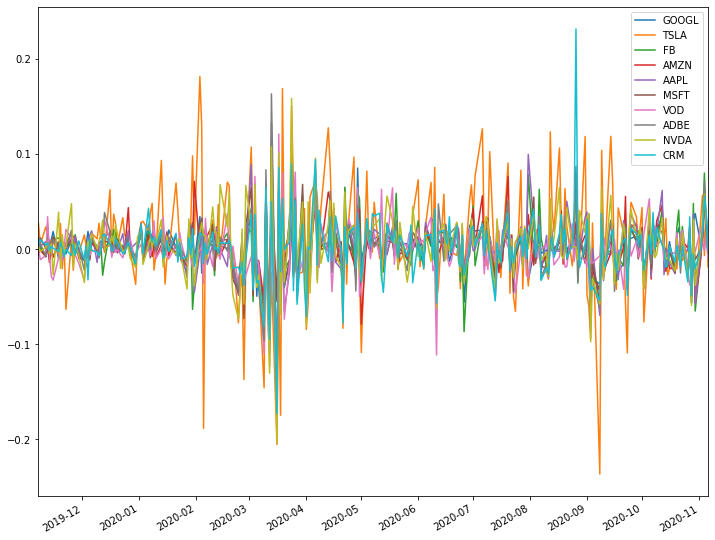# Portfolio Optimization in PythonLearn how you can construct diversified portfolios minimizing the risk using Python and SciPy. Use Python to automate optimization of portfolio using Modern Portfolio Theory.

We will show how you can build a diversified portfolio that satisfies specific constraints. For this tutorial, we will build a portfolio that minimizes the risk.

So the first thing to do is to get the stock prices programmatically using Python.

We will work with the package where you can install it using `pip install yfinance --upgrade --no-cache-dir` You will need to get the symbol of the stock. You can find the mapping between NASDAQ stocks and symbols in this csv file.

For this tutorial, we will assume that we are dealing with the following 10 stocks and we try to minimize the portfolio risk.

• Tesla with Symbol TSLA
• Amazon with Symbol AMZN
• Apple with Symbol AAPL
• Microsoft with Symbol MSFT
• Vodafone with Symbol VOD
• NVIDIA with Symbol NVDA
• Salesforce with Symbol CRM

``````import pandas as pd
import numpy as np
import yfinance as yf
from scipy.optimize import minimize
import matplotlib.pyplot as plt
%matplotlib inline
symbols = ['GOOGL', 'TSLA', 'FB', 'AMZN', 'AAPL', 'MSFT', 'VOD',  'ADBE', 'NVDA', 'CRM' ]
all_stocks = pd.DataFrame()
for symbol in symbols:
start='2019-11-07',
end='2020-11-07',
progress=False)['Close']
all_stocks = pd.concat([all_stocks, tmp_close], axis=1)
all_stocks.columns=symbols
all_stocks``````## Get the Log Returns

We will use the log returns or continuously compounded return. Let’s calculate them in Python.

``returns = np.log(all_stocks/all_stocks.shift(1)).dropna(how="any") returns````returns.plot(figsize=(12,10))``## Get the Mean Returns

We can get the mean returns of every stock as well as the average of all of them.

``````## mean daily returns per stock
returns.mean()

GOOGL    0.001224
TSLA     0.007448
FB       0.001685
AMZN     0.002419
AAPL     0.002422
MSFT     0.001740
VOD     -0.001583
NVDA     0.004077
CRM      0.001948
dtype: float64
## mean daily returns of all stocks
returns.mean().mean()
0.0023526909011353354``````

## Minimize the Risk of the Portfolio

Our goal is to construct a portfolio from those 10 stocks with the following constraints:

• The Expected daily return is higher than the average of all of them, i.e. greater than 0.003
• There is no short selling, i.e. we only buy stocks, so the sum of the weights of all stocks will ad up to 1
• Every stock can get a weight from 0 to 1, i.e. we can even build a portfolio of only one stock, or we can exclude some stocks.

Finally, our objective is to** minimize the variance (i.e. risk) of the portfolio**. You can find a nice explanation on this blog of how you can calculate the variance of the portfolio using matrix operations.

## Basic Data Types in Python | Python Web Development For Beginners

In the programming world, Data types play an important role. Each Variable is stored in different data types and responsible for various functions. Python had two different objects, and They are mutable and immutable objects.

## Hire Python Developers

Are you looking for experienced, reliable, and qualified Python developers? If yes, you have reached the right place. At **[HourlyDeveloper.io](https://hourlydeveloper.io/ "HourlyDeveloper.io")**, our full-stack Python development services...

## Applied Data Science with Python Certification Training Course -IgmGuru

Master Applied Data Science with Python and get noticed by the top Hiring Companies with IgmGuru's Data Science with Python Certification Program. Enroll Now

## Hire Python Developers India

Looking to build robust, scalable, and dynamic responsive websites and applications in Python? At **[HourlyDeveloper.io](https://hourlydeveloper.io/ "HourlyDeveloper.io")**, we constantly endeavor to give you exactly what you need. If you need to...

## Data Science With Python | Python For Data Science | Data Science For Beginners

This Data Science with Python Tutorial will help you understand what is Data Science, basics of Python for data analysis, why learn Python, how to install Python, Python libraries for data analysis, exploratory analysis using Pandas, introduction to series and dataframe, loan prediction problem, data wrangling using Pandas, building a predictive model using Scikit-Learn and implementing logistic regression model using Python.